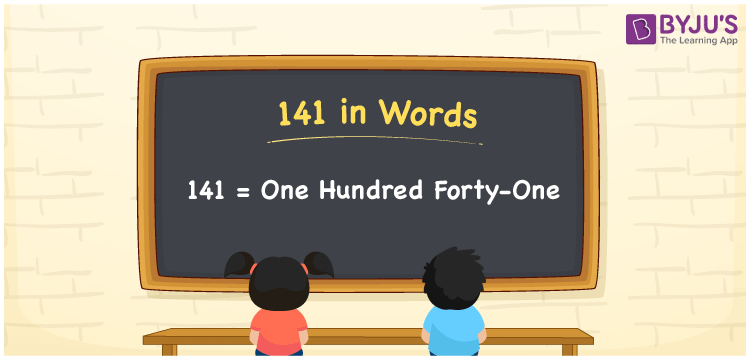# 141 in Words

We can write the number 141 in words as One hundred forty-one. If you marked Rs. 141, on a flashcard, ask your friend to read the same then, your friend may read it as, “One hundred forty-one rupees”. Thus, to spell the given number or express the given numerical amount, we can use the word form of that particular number. In this article, you will understand how to derive the cardinal number 141 in word form using a place value chart.

 141 in words One hundred forty-one One hundred forty-one in Numbers 141

## 141 in English Words

We generally use the English alphabet to express numbers in words. So, we spell 141 in English words as “One hundred forty-one”.## How to Write 141 in Words?

The number 141 has three digits so, we need to make a place value chart such that place values for all the three digits are assigned. This can be done as follows.

 Hundreds Tens Ones 1 4 1

Here, ones = 1, tens = 4, hundreds = 1

The above digits can be expanded based on their place values as:

1 × Hundred + 4 × Ten + 1 × One

= 1 × 100 + 4 × 10 + 1 × 1

= 100 + 40 + 1

= 100 + 41

= One hundred + Forty-one

= One hundred forty-one

Therefore, 141 in words = One hundred forty-one

141 is a natural number that precedes 142 and succeeds 140.

141 in words – One hundred forty-one

Is 141 an even number? – No

Is 141 an odd number? – Yes

Is 141 a prime number? – No

Is 141 a composite number? – Yes

Is 141 a perfect square number? – No

Is 141 a perfect cube number? – No

## Related Articles

### 141 to 150 in Words

 141 in words = One hundred forty-one 146 in words = One hundred forty-six 142 in words = One hundred forty-two 147 in words = One hundred forty-seven 143 in words = One hundred forty-three 148 in words = One hundred forty-eight 144 in words = One hundred forty-four 149 in words = One hundred forty-nine 145 in words = One hundred forty-five 150 in words = One hundred fifty

## Frequently Asked Questions on 141 in Words

Q1

### How do you write 141 in words?

Generally, we can write 141 in words as One hundred forty-one.
Q2

### What is the spelling of 141?

The spelling of 141 in English words is One hundred forty-one.
Q3

### How to write Rs. 141 in words on a cheque?

On a cheque, we can write Rs. 141 in words as “One hundred forty-one rupees only”.